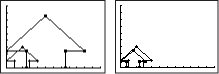# Transition Mathematics

Main goal: The main goal of Transition Mathematics is to act as a stepping-stone between the processes learned in Pre-Transition Mathematics or Everyday Mathematics 6 to the material presented in UCSMP Algebra and UCSMP Geometry. Transition Mathematics incorporates applied arithmetic, algebra, and geometry; and connects all these areas to measurement, probability, and statistics.

Main theme I: Arithmetic skills and concepts are reinforced by continuous instruction in the uses of the four basic operations of addition, subtraction, multiplication, and division. Basic skills and number sense practice are reinforced by applications and the conversions among decimals, fractions, and percents, with both positive and negative numbers.Picturing multiplication by 2.5 and by 0.8

Main theme II: The algebra in Transition Mathematics begins with the uses of variables in formulas, as pattern-generalizers, and as unknowns in solving problems. Graphing lines in the coordinate plane and the solving of linear equations and inequalities are developed.

Main theme III: The geometry in Transition Mathematics includes the use of transformations to demonstrate congruence, similarity, symmetry, and tessellations. Length, perimeter, area, and volume are studied as general concepts and with specific attention to common two- and three-dimensional figures. Drawing and constructions with and without the use of technology are both strongly encouraged throughout the text.

Some distinctive lessons: Graphing data on a calculator (1-10); Three little words: always, sometimes, never (4-1); Tessellations (6-4); How changing dimensions affects volume (11-10).

CCSS Lessons

Comparisons between this and earlier editions: The reality orientation of the material and the overall approach of this groundbreaking book remain. Some of the content in the first and second editions of Transition Mathematics has been moved to Pre-Transition Mathematics due to (1) the existence of Everyday Mathematics and the general increase in the performance of students coming into middle school, (2) increased expectations for the performance of all students in both middle and high schools and the concomitant increased levels of testing, and (3) recommendations for more algebra and geometry in middle school courses preceding year-long courses in algebra and geometry. Calculators with graphing and list features are introduced early as pattern-fitting and problem-solving tools. Spreadsheets and dynamic geometry systems are found in activities throughout the materials. Students are engaged and learning is reinforced with the use of games.

### Contact

UCSMP
1427 East 60th Street
Chicago, IL 60637
T: 773-702-1130
F: 773-834-4665
ucsmp@uchicago.edu